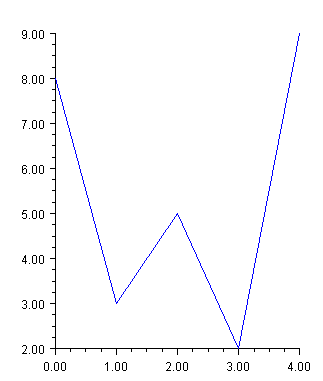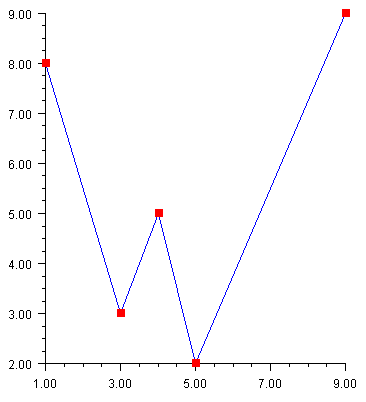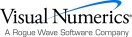## Line Plot

A line plot consists of points by lines. The lines can be formatted using the Line Attributes.

### Simple Line Plot

This example shows a simple line plot. The Data node is created using an array of y-values. The x-values default to 0, 1, …. The DataType attribute is set to DATA_TYPE_LINE to make this a line chart. The look of the line is controlled by the line attributes. Here the LineColor attribute is set to blue.using Imsl.Chart2D;

using System.Drawing;

public class SampleSimpleLine : FrameChart {

public SampleSimpleLine() {

Chart chart = this.Chart;

AxisXY axis = new AxisXY(chart);

double[] y = new double[] {8, 3, 5, 2, 9};

Data data1 = new Data(axis, y);

data1.DataType = Data.DATA_TYPE_LINE;

data1.LineColor = Color.Blue;

}

public static void Main(string[] argv) {

System.Windows.Forms.Application.Run(new SampleSimpleLine());

}

}

### Mixed Line and Marker Plot

The DataType attribute can be set using “or” syntax to combine types. In this example, it is set to DATA_TYPE_LINE | DATA_TYPE_MARKER. This example also explicitly sets both the x-value and the y-value of the data points. Note that the x-values do not have to be uniformly spaced.using Imsl.Chart2D;

using System.Drawing;

public class SampleLineMarker : FrameChart {

public SampleLineMarker() {

Chart chart = this.Chart;

AxisXY axis = new AxisXY(chart);

double[] x = new double[] {1, 3, 4, 5, 9};

double[] y = new double[] {8, 3, 5, 2, 9};

Data data1 = new Data(axis, x, y);

data1.DataType = Data.DATA_TYPE_LINE | Data.DATA_TYPE_MARKER;

data1.LineColor = Color.Blue;

data1.MarkerColor = Color.Red;

data1.MarkerType = Data.MARKER_TYPE_FILLED_SQUARE;

}

public static void Main(string[] argv) {

System.Windows.Forms.Application.Run(new SampleLineMarker());

}

}Visual Numerics - Developers of IMSL and PV-WAVEhttp://www.vni.com/PHONE: 713.784.3131FAX:713.781.9260